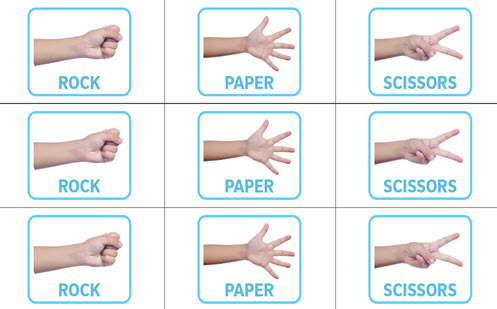Go to website

# reSolve: Probability

This sequence of four lessons explores probability in real-world situations including advertising, games and population sampling. Students calculate probabilities, represent probabilities as fractions, decimals and percentages, perform chance experiments with small and large sample sizes and graph their results, examine the language of probability including the concept of 'luck', and learn about population sampling by conducting their own sampling experiments. Each lesson is outlined in detail including curriculum links, vocabulary, materials needed, sample answers, discussion points and student resources such as spreadsheets. This sequence is part of the reSolve: Mathematics by Inquiry program.

Year level(s) Year 6
Audience Teacher
Purpose Teaching resource
Teaching strategies and pedagogical approaches Mathematics investigation
Keywords sampling, expected frequency, statistical frequency, observations

## Curriculum alignment

Curriculum connections Numeracy
Strand and focus Build understanding, Apply understanding, Probability
Topics Chance and probability
AC: Mathematics (V9.0) content descriptions
AC9M6P01

Recognise that probabilities lie on numerical scales of 0 - 1 or 0% - 100% and use estimation to assign probabilities that events occur in a given context, using common fractions, percentages and decimals

AC9M6P02

Conduct repeated chance experiments and run simulations with an increasing number of trials using digital tools; compare observations with expected results and discuss the effect on variation of increasing the number of trials

Numeracy progression Proportional thinking (P1)
Understanding chance (P4)